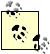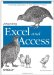# 5.2. Other Excel Objects

 < Day Day Up >

Programming with the Excel object model is generally intuitive if you are familiar with Excel. For example, cell, range, columns, rows, etc. are all referred to by their common names. While the syntax might not be intuitive, the Visual Basic Editor helps you write it (provided that you use early binding). For these examples, assume that you used the earlier code to create an Excel application, workbook, and worksheet. Let's say that you want to put the text "Employee Data" in cell B4 and you want it to be bold with a border around it. You also want that column width to fit the text. You could do this in a number of ways.

#### 5.2.1. The Range Property and Object

Using a Range object and the Range property of a worksheet is probably the most straightforward way of doing this task. Use the Dim statement to create a variable for an Excel range, or simply use xlWs.Range("B4").value = "Employee Data". Your choice depends on what you need to do with the range. Since we have a stated goal of formatting this range, I suggest using the Range object as a variable. The code to do this in Access is in Example 5-6.

##### Example 5-6. Using the range object
` Public Sub GetExcel( ) Dim xlApp As Excel.Application Dim xlWb As Excel.Workbook Dim xlWs As Excel.Worksheet Dim xlRng As Excel.Range Set xlApp = New Excel.Application xlApp.Visible = True Set xlWb = xlApp.Workbooks.Add Set xlWs = xlWb.Worksheets.Add xlWs.Name = "MyNewWorksheet" Set xlRng = xlWs.Range("B4") With xlRng   .Value = "Employee Data"   .Font.Bold = True   .BorderAround xlContinuous, xlThick, 5 End With xlWs.Columns("B").AutoFit End Sub `

As previously mentioned, there are several ways to accomplish this. Instead of the Range object, you could also use either the Cells property or Offset to refer to a range.

#### 5.2.2. The Cells Property

When you know the range ahead of time, it is probably easier to refer to it as it is above. However, if you are writing to multiple cells or if you have variables that hold both row and column numbers, it is very difficult to use the Range property. To do so, you need to create some type of lookup to show that the third column is column C, and you need to create a string and concatenate the C with the row number. This difficulty is solved very easily by using the Cells property, which returns a Range object that you can handle as in the previous example. The syntax of the Cells property is Worksheetobject.Cells(Row Number, Column Number). You can also continue using the same properties and methods as you would a Range object, but you will not get help while typing it. For this reason, I generally use the Cells property with a Range object unless I am writing to the Value property.

Looking at the Example 5-6, you can change two lines to use the Cells property. In Example 5-7, changes have been made: first, the cells property is used to refer to cell B4 row 4 and column 2; and second, for the column's autofit, I refer to the column by number.

##### Example 5-7. Using the cells property to select a range
` Public Sub GetExcel( ) Dim xlApp As Excel.Application Dim xlWb As Excel.Workbook Dim xlWs As Excel.Worksheet Dim xlRng As Excel.Range Set xlApp = New Excel.Application xlApp.Visible = True Set xlWb = xlApp.Workbooks.Add Set xlWs = xlWb.Worksheets.Add xlWs.Name = "MyNewWorksheet" Set xlRng = xlWs.Cells(4, 2) With xlRng   .Value = "Employee Data"   .Font.Bold = True   .BorderAround xlContinuous, xlThick, 5 End With xlWs.Columns(2).AutoFit End Sub `

#### 5.2.3. Using Offset

When you have already referred to a range, and you want to move a certain number of rows and columns, you can use the Offset method of the Range object to return a new Range object. The syntax of the Offset method is Rangeobject.Offset(Row Offset, Column Offset), which returns a range. In the next example, the range object is set to A1. To move to B4, offset the row by 3 and the column by 1. This is useful when you do not have a variable for the column or row and you want to continue moving by a certain number of rows and/or columns. I am less likely to use this than the Cells property, but I am more likely to use this than a reference directly to a range. Understanding this property is important for later possible uses of R1C1 notation when writing formulas with VBA. The other change in Example 5-8 is that I refer to the column to auto fit by using the Column property of the Range object. The Row property can also be referenced if you need to know which row a range refers to.

##### Example 5-8. Using Offset to select a range
` Public Sub GetExcel2( ) Dim xlApp As Excel.Application Dim xlWb As Excel.Workbook Dim xlWs As Excel.Worksheet Dim xlRng As Excel.Range Set xlApp = New Excel.Application xlApp.Visible = True Set xlWb = xlApp.Workbooks.Add Set xlWs = xlWb.Worksheets.Add xlWs.Name = "MyNewWorksheet" Set xlRng = xlWs.Range("A1") Set xlRng = xlRng.Offset(3, 1) With xlRng   .Value = "Employee Data"   .Font.Bold = True   .BorderAround xlContinuous, xlThick, 5 End With xlWs.Columns(xlRng.Column).AutoFit End Sub `

Many other Excel objects will be used throughout the book. Understanding the basic objects presented here and their common properties and methods is a good basis to begin automation. The difficulty with automation is that you can do the same thing a variety of ways. For the most part, there are no right answers as to which is the best way. Usually, the objects, methods, and properties used are determined by what the programmer is familiar with. I show multiple methods so that you do not get stuck using a more difficult property.If you want to auto fit all of the columns on a worksheet, use Worksheetobject.Columns.AutoFit instead of referring to each column individually.

 < Day Day Up >Integrating Excel and Access
ISBN: 0596009739
EAN: 2147483647
Year: 2005
Pages: 132

Similar book on Amazon